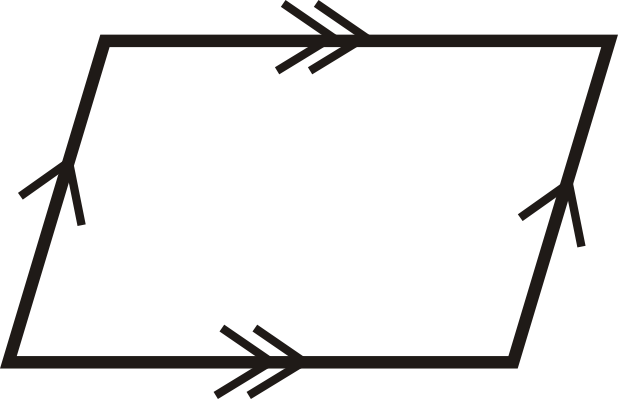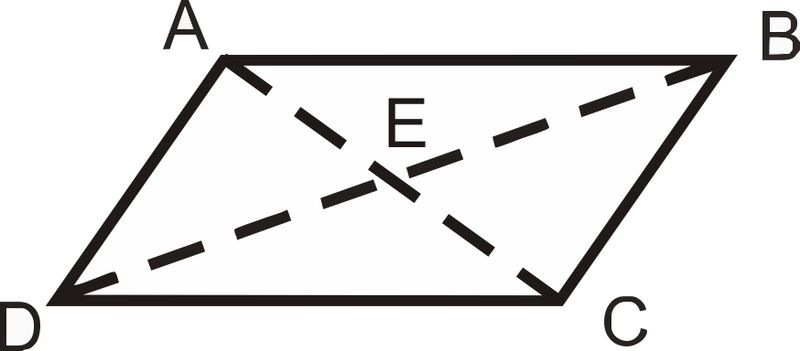# 2.16: Parallelogram Proofs

•• Contributed by CK12
• CK12

Apply theorems to show if a quadrilateral has two pairs of parallel sides.

Recall that a parallelogram is a quadrilateral with two pairs of parallel sides. Even if a quadrilateral is not marked with having two pairs of sides, it still might be a parallelogram. The following is a list of theorems that will help you decide if a quadrilateral is a parallelogram or not.

1. Opposite Sides Theorem Converse: If both pairs of opposite sides of a quadrilateral are congruent, then the figure is a parallelogram.

IfFigure $$\PageIndex{1}$$

thenFigure $$\PageIndex{2}$$

2. Opposite Angles Theorem Converse: If both pairs of opposite angles of a quadrilateral are congruent, then the figure is a parallelogram.

IfFigure $$\PageIndex{3}$$

thenFigure $$\PageIndex{4}$$

3. Parallelogram Diagonals Theorem Converse: If the diagonals of a quadrilateral bisect each other, then the figure is a parallelogram.

IfFigure $$\PageIndex{5}$$

thenFigure $$\PageIndex{6}$$

4. Parallel Congruent Sides Theorem: If a quadrilateral has one set of parallel lines that are also congruent, then it is a parallelogram.

IfFigure $$\PageIndex{7}$$

thenFigure $$\PageIndex{8}$$

You can use any of the above theorems to help show that a quadrilateral is a parallelogram. If you are working in the x−y plane, you might need to know the formulas shown below to help you use the theorems.

• The Slope Formula, $$\dfrac{y_2−y_1}{x_2−x_1}$$. (Remember that if slopes are the same then lines are parallel).
• The Distance Formula, $$\sqrt{(x_2−x_1)^2+(y_2−y_1)^2}$$. (This will help you to show that two sides are congruent).
• The Midpoint Formula, $$( \dfrac{x_1+x_2}{2}, \dfrac{y_1+y_2}{2} )$$. (If the midpoints of the diagonals are the same then the diagonals bisect each other).

What if you were given four pairs of coordinates that form a quadrilateral? How could you determine if that quadrilateral is a parallelogram?

Example $$\PageIndex{1}$$

Prove the Parallel Congruent Sides Theorem.Figure $$\PageIndex{9}$$

Given: $$\overline{AB}\parallel\overline{DC}$$, and $$\overline{AB}\cong \overline{DC}$$

Prove: $$ABCD$$ is a parallelogram

Solution

Statement Reason
1. $$\overline{AB}\parallel\overline{DC}$$, and $$\overline{AB}\cong \overline{DC}$$ 1. Given
2. $$\angle ABD\cong \angle BDC$$ 2. Alternate Interior Angles
3. $$\overline{DB}\cong \overline{DB}$$ 3. Reflexive $$PoC$$
4. $$\Delta ABD\cong \Delta CDB$$ 4. SAS
5. $$\overline{AD}\cong \overline{BC}$$\) 5. CPCTC
6. $$ABCD$$ is a parallelogram 6. Opposite Sides Converse

Example $$\PageIndex{2}$$

What value of $$x$$ would make $$ABCD$$ a parallelogram?Figure $$\PageIndex{10}$$

Solution

$$\overline{AB}\parallel\overline{DC}$$. By the Parallel Congruent Sides Theorem, $$ABCD$$ would be a parallelogram if $$AB=DC$$.

\begin{align*} 5x−8 &=2x+13 \\ 3x &=21 \\ x &=7 \end{align*}

Example $$\PageIndex{3}$$

Prove the Opposite Sides Theorem Converse.Figure $$\PageIndex{11}$$

Given: $$\overline{AB}\cong \overline{DC}$$, $$\overline{AD}\cong \overline{BC}$$

Prove: $$ABCD$$ is a parallelogram

Solution

Statement Reason
1. $$\overline{AB}\cong \overline{DC}$$,$$\overline{AD}\cong \overline{BC}$$ 1.Given
2. $$\overline{DB}\cong \overline{DB}$$ 2. Reflexive $$PoC$$
3. $$\Delta ABD\cong \Delta CDB$$ 3. SSS
4. $$\angle ABD\cong \angle BDC$$,$$\angle ADB\cong \angle DBC$$ 4. $$CPCTC$$
5. $$\overline{AB}\parallel\overline{DC}$$,$$\overline{AD}\parallel\overline{BC}$$ 5. Alternate Interior Angles Converse
6. $$ABCD$$ is a parallelogram 6. Definition of a parallelogram

Example $$\PageIndex{4}$$

Is quadrilateral $$EFGH$$ a parallelogram? How do you know?Figure $$\PageIndex{12}$$

Solution

By the Opposite Angles Theorem Converse, $$EFGH$$ is a parallelogram.

$$EFGH$$ is not a parallelogram because the diagonals do not bisect each other.

Example $$\PageIndex{5}$$

Is the quadrilateral $$ABCD$$ a parallelogram?Figure $$\PageIndex{13}$$

Solution

Let’s use the Parallel Congruent Sides Theorem to see if $$ABCD$$ is a parallelogram. First, find the length of AB and CD using the distance formula.

\begin{align*} AB &=\sqrt{(−1−3)^2+(5−3)^2} & CD &=\sqrt{(2−6)^2+(−2+4)^2} \\ &=\sqrt{(−4)^{2}+2^2} & &=\sqrt{(−4)^2+2^2} \\ &=\sqrt{16+4}=\sqrt{20} & & =\sqrt{16+4}=\sqrt{20} \end{align*}

Next find the slopes to check if the lines are parallel.

\begin{align*} Slope \: AB =\dfrac{5−3}{−1−3} =\dfrac{2}{−4} &=−\dfrac{1}{2} &Slope \: CD=\dfrac{−2+4}{2−6}=\dfrac{2}{−4}=−\dfrac{1}{2} \end{align*}

$$AB=CD$$ and the slopes are the same (implying that the lines are parallel), so $$ABCD$$ is a parallelogram.

## Review

For questions 1-12, determine if the quadrilaterals are parallelograms.

1.Figure $$\PageIndex{14}$$
2.Figure $$\PageIndex{15}$$
3.Figure $$\PageIndex{16}$$
4.Figure $$\PageIndex{17}$$
5.Figure $$\PageIndex{18}$$
6.Figure $$\PageIndex{19}$$
7.Figure $$\PageIndex{20}$$
8.Figure $$\PageIndex{21}$$
9.Figure $$\PageIndex{22}$$
10.Figure $$\PageIndex{23}$$
11.Figure $$\PageIndex{24}$$
12.Figure $$\PageIndex{25}$$

For questions 13-18, determine the value of $$x$$ and $$y$$ that would make the quadrilateral a parallelogram.

1.Figure $$\PageIndex{26}$$
2.Figure $$\PageIndex{27}$$
3.Figure $$\PageIndex{28}$$
4.Figure $$\PageIndex{29}$$
5.Figure $$\PageIndex{30}$$
6.Figure $$\PageIndex{31}$$

For questions 19-22, determine if $$ABCD$$ is a parallelogram.

1. $$A(8,−1)$$, $$B(6,5)$$, $$C(−7,2)$$, $$D(−5,−4)$$
2. $$A(−5,8)$$, $$B(−2,9)$$, $$C(3,4)$$, $$D(0,3)$$
3. $$A(−2,6)$$, $$B(4,−4)$$, $$C(13,−7)$$, $$D(4,−10)$$
4. $$A(−9,−1)$$, $$B(−7,5)$$, $$C(3,8)$$, $$D(1,2)$$

Fill in the blanks in the proofs below.

1. Opposite Angles Theorem ConverseFigure $$\PageIndex{32}$$

Given: $$\angle A\cong \angle C$$, $$\angle D\cong \angle B$$

Prove: $$ABCD$$ is a parallelogram

Statement Reason
1. 1.
2. $$m\angle A=m\angle C$$, $$m\angle D=m\angle B$$ 2.
3. 3. Definition of a quadrilateral
4. $$m\angle A+m\angle A+m\angle B+m\angle B=360^{\circ}$$ 4.
5. 5. Combine Like Terms
6. 6. Division $$PoE$$
7. $$\angle A$$ and $$\angle B$$ are supplementary $$\angle A$$ and $$\angle D$$ are supplementary 7.
8. 8. Consecutive Interior Angles Converse
9. $$ABCD$$ is a parallelogram 9.
1. Parallelogram Diagonals Theorem ConverseFigure $$\PageIndex{33}$$

Given: $$\overline{AE}\cong \overline{EC}$$, $$\overline{DE}\cong \overline{EB}$$

Prove: $$ABCD$$ is a parallelogram

Statement Reason
1. 1.
2. 2. Vertical Angles Theorem

3. $$\Delta AED \cong \Delta CEB$$

$$\Delta AEB\cong \Delta CED$$

3.
4. 4.
5. $$ABCD$$ is a parallelogram 5.
1. Given: $$\angle ADB\cong \angle CBD$$, $$\overline{AD}\cong \overline{BC}$$

Prove: $$ABCD$$ is a parallelogramFigure $$\PageIndex{34}$$
Statement Reason
1. 1.
2. $$\overline{AD}\parallel\overline{BC}$$ 2.
3. $$ABCD$$ is a parallelogram 3.

Interactive Element

Video: Proving a Quadrilateral is a Parallelogram Principles - Basic

Activities: Quadrilaterals that are Parallelograms Discussion Questions

Study Aids: Parallelograms Study Guide

Practice: Parallelogram Proofs

Real World: Quadrilaterals That Are Parallelograms